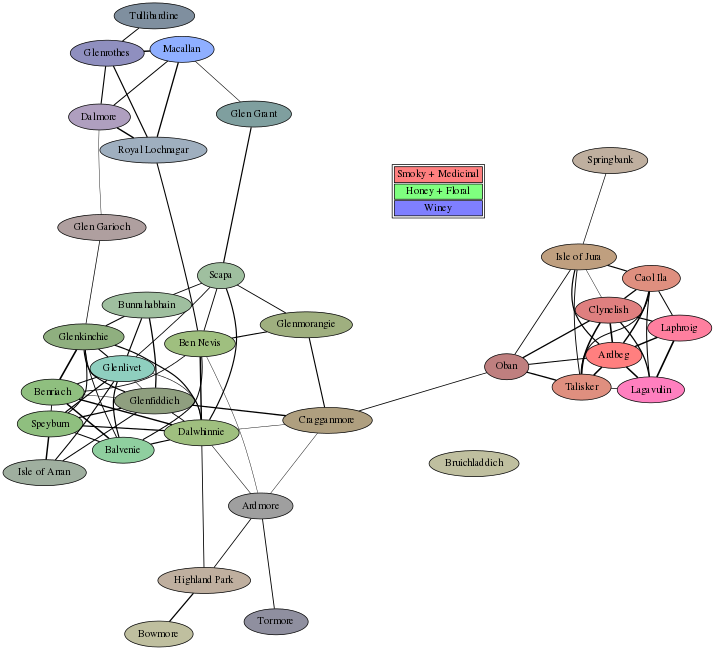# Scotch Graph¶

There is a data set of 86 scotch distilleries that tends to make its way around the internet in various forms, showing up in numerous plots and clustered charts. Nobody seems to know how the data was gathered, or which bottling was used for which distilliry, but the data appears to be accurate. More importantly: it is freely available, and thus perfect for messing around with.

## Data Import¶

Step 1 is loading the data. Since the plan was to plot each distillery as a node in an undirected graph ), I cut about 50 distilleries from the CSV for legibility. Running these steps for all 86 data points produces a crowded mess (available at the bottom of this page).

Loading a CSV file into a Pandas DataFrame is ridiculously easy; the "hard" part is fixing up the indices:

In :
import pandas as pd

re_idx = [i.replace(' ', '_') for i in scotch.Distillery]
scotch = scotch[scotch.columns[2:-3]].set_index(pd.Series(re_idx))


Out:
Body Sweetness Smoky Medicinal Tobacco Honey Spicy Winey Nutty Malty Fruity Floral
Ardbeg 4 1 4 4 0 0 2 0 1 2 1 0
Ardmore 2 2 2 0 0 1 1 1 2 3 1 1
Isle_of_Arran 2 3 1 1 0 1 1 1 0 1 1 2
Balvenie 3 2 1 0 0 3 2 1 0 2 2 2
Ben_Nevis 4 2 2 0 0 2 2 0 2 2 2 2

## Data Analysis¶

With a DataFrame full of data, we can rearrange it, correlate all the distilleries by flavor, and rearrange the data again, all in under 40 characters:

In :
sc = scotch.T.corr().unstack()


Out:
Ardbeg  Ardbeg           1.000000
Ardmore          0.231617
Isle_of_Arran    0.123130
Balvenie        -0.025788
Ben_Nevis        0.307534
dtype: float64

Next up is the fun part: using the calculated correlations to build a graph. Python has many options for using GraphViz, so naturally the easiest solution is to just generate a list raw GraphViz statements:

In :
from math import sqrt

def get_node(df, name):
s = df.loc[name]
r = 127+16*int(s['Smoky'] + s['Medicinal'])
g = 127+16*int(s['Honey'] + s['Floral'])
b = 127+32*int(s['Winey'])
return '{} [label="{}",style=filled,fillcolor="#{:0>2x}{:0>2x}{:0>2x}"];'.format(
name,      name.replace('_', ' '),      r,     g,     b
)

def get_edge(n1, n2, corr, thresh=0.65):
if corr > thresh and n1 < n2:
knorm = (corr - thresh) / (1.0 - thresh)
w = int(1000*knorm) + 1
p = 2*sqrt(knorm) + 0.5
return '{} -- {} [weight={},penwidth={}];'.format(
n1,   n2,        w,          p
)

graph_list = [
'graph scotch {',
'subgraph legend{',
'node [shape=plaintext];',
'label = "Legend";',
'all [label=<<table>',
'<tr><td bgcolor="#ff7f7f">Smoky + Medicinal</td></tr>',
'<tr><td bgcolor="#7fff7f">Honey + Floral</td></tr>',
'<tr><td bgcolor="#7f7fff">Winey</td></tr>',
'</table>>];',

'}']

for p, k in sc.iteritems():
if p == p:
graph_list += [get_node(scotch, p)]
else:
e = get_edge(p, p, k)
if e:
graph_list += [e]

graph_list += ['}']

graph_list[15:20]

Out:
['Ardbeg -- Laphroig [weight=561,penwidth=1.9968310955185609];',
'Ardbeg -- Oban [weight=204,penwidth=1.4022520211817107];',
'Ardbeg -- Talisker [weight=711,penwidth=2.1863140012360973];',
'Ardmore [label="Ardmore",style=filled,fillcolor="#9f9f9f"];',
'Ardmore -- Ben_Nevis [weight=2,penwidth=0.571204782182186];']

Note that the color of each node is determined by a linear combination of five flavors. The coloring choices were made manually, but they were informed by looking at what flavors correlated with each other (found via scotch.corr().unstack()). Edge width (i.e. penwidth) was set based on the correlation, such that distilleries with similar flavor profiles have thicker lines connecting them.

Now that we have a bunch of nodes and edges, we feed them to GraphVis (and then back into IPython, for display):

In :
import subprocess, io
from IPython.display import display, Image

gv_cmd = 'sfdp -Goverlap=prism -Gratio=0.5 -Gsplines=true -Gdpi=52 -GK=1.5 -Tpng'.split(' ')
inp = bytearray('\n'.join(graph_list), 'ascii')

subp = subprocess.Popen(gv_cmd, stdin=subprocess.PIPE, stdout=subprocess.PIPE, stderr=subprocess.PIPE)
(outp, err) = subp.communicate(inp)
subp.wait()

if subp.returncode == 0:
display(Image(outp))
else:
print(err)Also available as a PDF, or as a PDF with all distilleries.